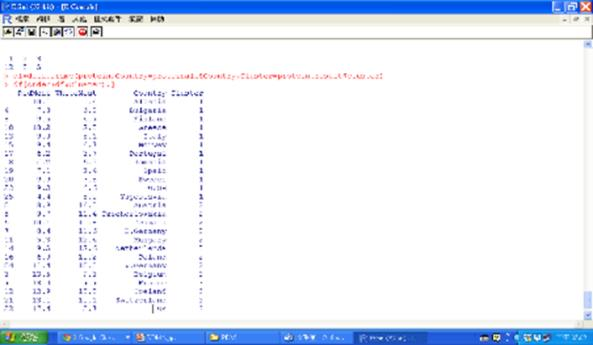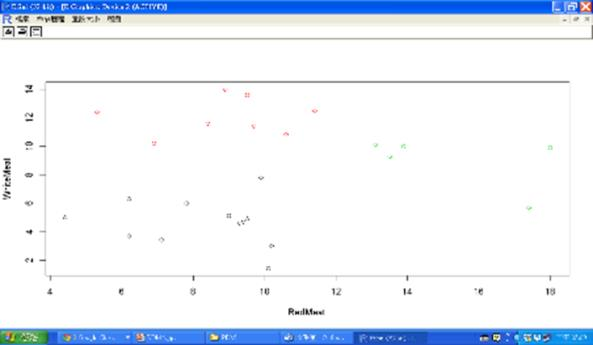﻿ R軟體資料探勘實務(下)—集群模型

# ntuccepaper2019

## 技術論壇

### 手機版選單

#### 字級

R軟體資料探勘實務(下)—集群模型
• 卷期：v0037
• 出版日期：2016-06-20

### 一. 鳶尾花資料集的各種集群模型

1. K-平均數(K-Means)集群
K-Means演算法是麥昆(J. B. MacQueen)於1967年正式發表，由於原理簡單、計算快速，很快就成為商用資料採礦軟體中的基本配備。它是屬於前 設式的集群演算法，也就是必須先設定集群的數量，然後根據該設定找出最 佳的集群結構。使同一集群內的資料物件相似性大，不同類別中的資料差異性大，也就是達到「組內同質，組間異質」的處理方式。
K平均數(K-means)是最受使用者歡迎以及最佳的集群分析法之一，屬 分割式分群法，分群方式是先將原始事物分為k個群體，計算某一資料點到集群中心之距離（或相適度），將其分配到最接近的群體，重新計算增加及減少資料點的集群中心，重複計算直至各資料點不必重新分配至其他集群為止。

#iris2=iris[,sapply(iris,is.numeric)]取所有數值資料的指令或者
iris2=iris[,-5]
set.seed(1234)
kmeans.result=kmeans(iris2,3)
kmeans.result
table(iris\$Species,kmeans.result\$cluster)
plot(iris2,col=kmeans.result\$cluster)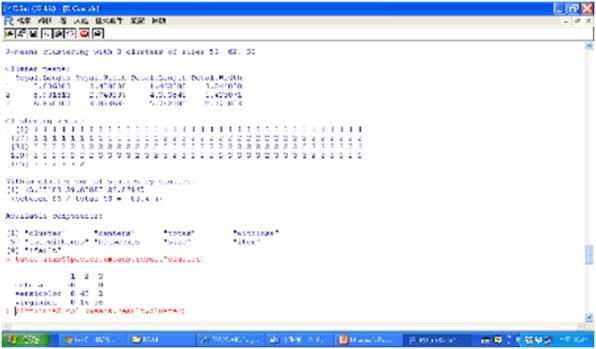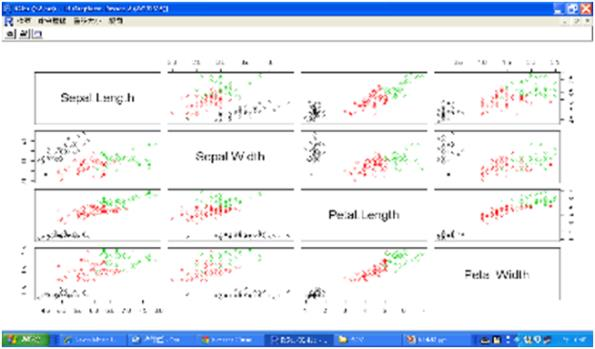#find 5 largest distances between objects and cluster centers
kmeans.result\$centers
centers=kmeans.result\$centers[kmeans.result\$cluster,]
distances=sqrt(rowSums((iris2-centers)^2))
outliers=order(distances,decreasing=T)[1:5]
outliers
iris2[outliers,]
plot(iris2[c("Sepal.Length", "Sepal.Width")], col=kmeans.result\$cluster)
points(kmeans.result\$centers[,c("Sepal.Length", "Sepal.Width")], col=1:3, pch=8, cex=2)
points(iris2[outliers,c("Sepal.Length", "Sepal.Width")], col=4, pch='+', cex=2)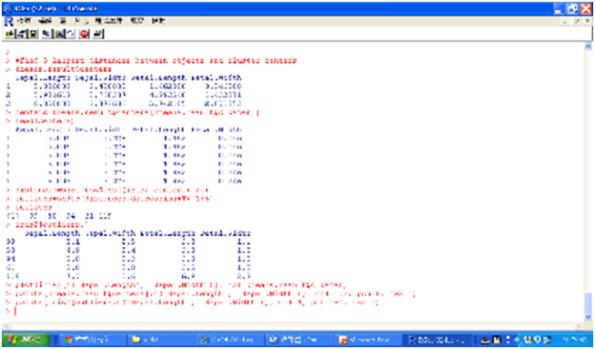2. K-物件(K-Medoids)集群
K-物件集群法是以集群中最具代表性的點Medoid作為集群中心，亦即 利用真正的物件代表集群。K-Medoids最經典演算法是分割環繞物件(PAM, Partitioning Around Medoids)，由Kaufman and Rousseeuw於1987年提出。 可使用cluster套件的pam函數，或是fpc套件的pamk函數，使用pamk函 數時，使用者不必決定分成幾組，但結果不一定理想。pamk程式碼如下：
iris2=iris[,-5]
library(fpc)
pamk.result=pamk(iris2)
pamk.result
table(iris\$Species, pamk.result\$pamobject\$clustering)
layout(matrix(c(1,2),1,2))
plot(pamk.result\$pamobject)
layout(matrix(1))Silhouett係數範圍在-1(非常差的模型)和1(非常好的模型)之間，圖中的Silhouette係數為(51*0.81+99*0.62)/150=0.69。library(NbClust)
iris2=iris[,-5]
result=NbClust(iris2,distance="euclidean",min.nc=2,max.nc=6, method="kmeans", index="all")
Result\$Best.partition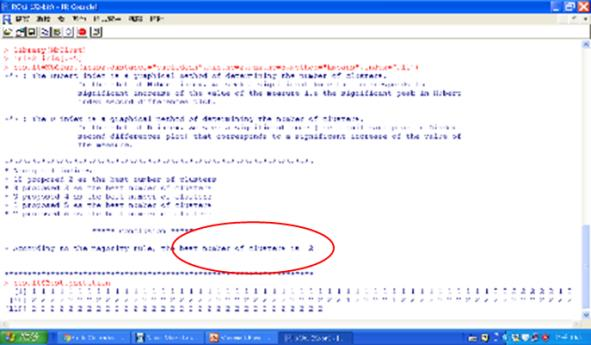3. 階層式(Hierarchical)集群

method=”average“，平均距離的平均連鎖法
method=”simple“，最小距離的單一連鎖法
method=”complete“，最大距離的完全連鎖法
method=”ward.D“或 "ward.D2"，Ward的最小變異法

iris2=iris[,-5]
index=sample(1:nrow(iris2),40) #抽取40筆
irissample=iris2[index,]
#dist函數 distance matrix compute
hclust.result=hclust(dist(irissample),method= “ward.D2”)
hclust.result
plot(hclust.result,labels=iris\$Species[index])
rect.hclust(hclust.result,k=3,border="red")
groups=cutree(hclust.result,k=3)
table(iris\$Species[index],groups)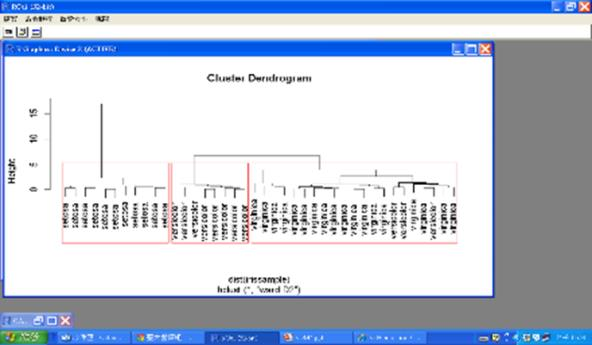4. 密度基礎(Density-based)集群
fpc套件的dbscan演算法可提供數值資料的密度基礎集群，dbscan有兩個重要參數，即可達區域eps，定義鄰近地區的大小，以及可達區域的最小點MinPts。如果某個點鄰近地區的點數大於minPts，那麼這個點就是一個密度點，所有從這個點可達的鄰近地區的點就被分成同一個集群。dbscan的程式碼如下：
iris2=iris[,-5]
library(fpc)
dbscan.result=dbscan(iris2,eps=0.42,MinPts=5)
dbscan.result
table(iris\$Species, dbscan.result\$cluster)
plot(dbscan.result,iris2)
plotcluster(iris2,dbscan.result\$cluster)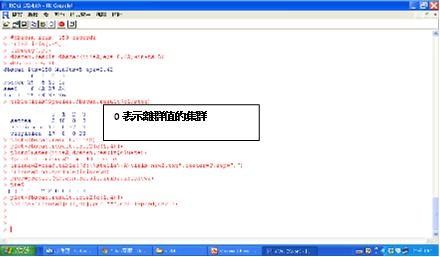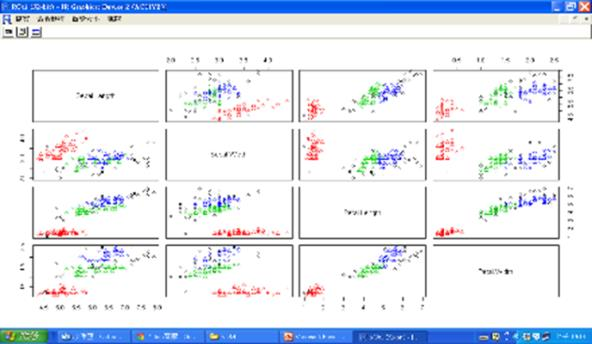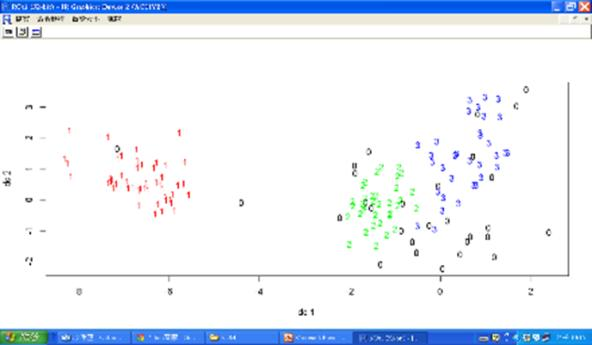### 二. 動物園的動物集群範例

1. 動物的名字(animal name)：唯一值
2. 是否具有毛髮(hair)：Boolean值
3. 身體是否有羽毛(feathers)：Boolean值
4. 是否會下蛋(eggs)：Boolean值
5. 是否會產奶(milk)：Boolean值
6. 是否在空中(airborne)：Boolean值
7. 是否在水中(aquatic)：Boolean值
8. 是否會獵食(predator)：Boolean值
9. 是否有牙齒(toothed)：Boolean值
10. 是否有骨幹(backbone)：Boolean值
11. 是否會呼吸(breathes)：Boolean值
12. 是否有毒(venomous)：Boolean值
13. 是否有鰭(fins)：Boolean值
14. 腿的數量(legs)：{0,2,4,5,6,8}集合的數字
15. 是否有尾巴(tail)：Boolean值
16. 是否被馴化(domestic)：Boolean值
17. 是否屬貓科(catsize)：Boolean值
18. 類型(type)：在[1,7]範圍內的整數值

zoo=na.exclude(zoo)
#kzoo=zoo[,sapply(zoo,is.numeric)] 取所有數值資料
kzoo=zoo[,-c(1,18)]
set.seed(777)
kresult=kmeans(kzoo,7)
kresult
table(kresult\$cluster)
table(zoo\$type,kresult\$cluster)
kresult\$size
pie(kresult\$size)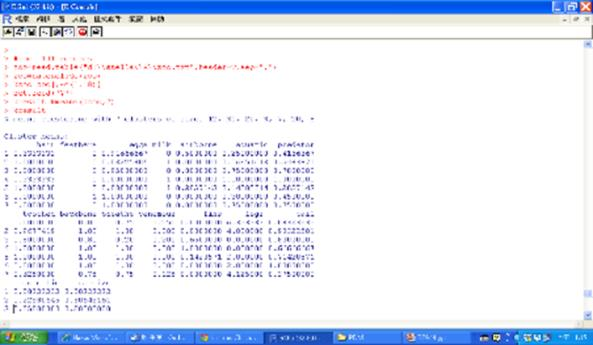### 三. 電信公司的客戶集群範例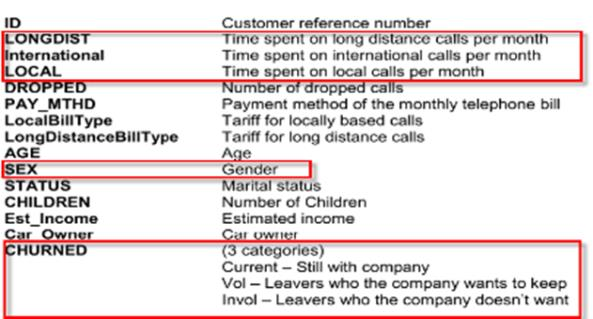churnall=na.exclude(churnall)
churn=churnall[,c(2:4)]
churn.result=kmeans(churn,5)
table(churn.result\$cluster)
pie(table(churn.result\$cluster))
table(churnall\$CHURNED,churn.result\$cluster)
barplot(table(churnall\$CHURNED,churn.result\$cluster), col=2:4)
legend(0,600,c("Current","Invol","Vol"),col=2:4,pch=15)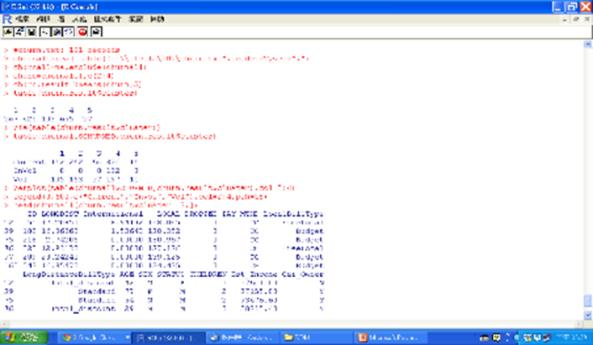### 四. 蛋白質攝取的集群分析範例(proteinall=na.exclude(proteinall))
protein=proteinall[,c(2:3)]
library(fpc)
protein.result=pamk(protein)
protein.result\$nc
protein.result=kmeans(protein,3) #nc
table(protein.result\$cluster)
df=data.frame(protein,Country=proteinall\$Country, Cluster=protein.result\$cluster)
df[order(df\$Cluster),]
plot(protein,col=df\$Cluster)